The flow in which flow parameters such as velocity, pressure and density of a fluid flow are dependent upon time is called unsteady flow.

In pipe lines when the valves are operated then the flow parameters changes with respect to time and its state becomes unsteady.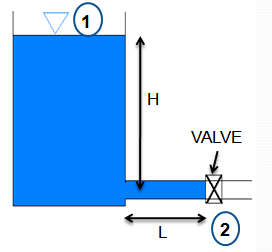Transient Time
• Transient time is important to know so that the closing or opening times of the valves can be adjusted.
• Due to unsteadiness high and low pressure waves develop in the pipes.
• If not controlled the pipes can burst due to high pressure or collapse due to low pressure.
• For Transient Time Writing Energy Equation Between Point 1 And 2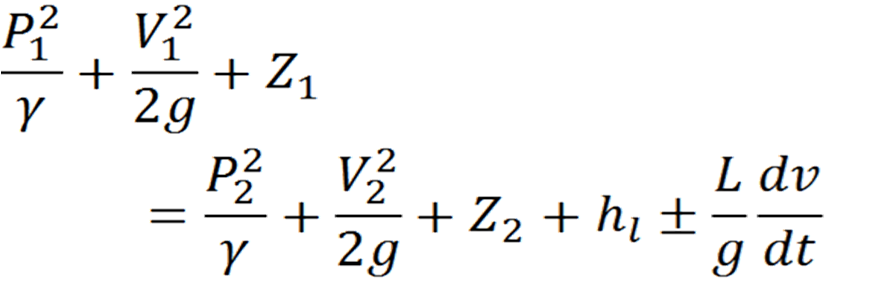Equation.1
• If there is atmospheric pressure at both sections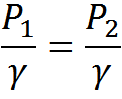• If datum is at pipe central line then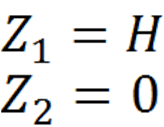• The head loss is due to
1. Separation losses
2. Pipe friction
• Unsteady flow energy balance also known as accelerative head is  given by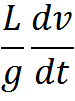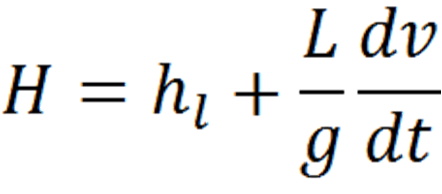Equation.2
• The energy equation becomes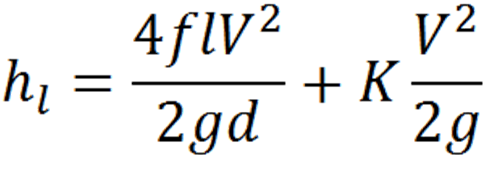Equation.3
f = pipe friction factor
K = Separation Losses
• Finally Equation 1 becomes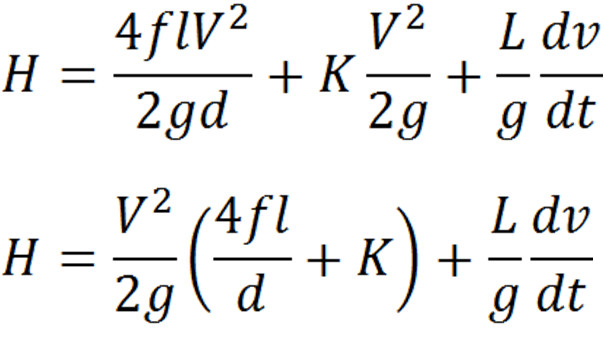Equation.4
• When valve is opened the head H is used to accelerate the flow at such a value at which the head is balanced by frictional and minor losses.
• The energy equation can be written as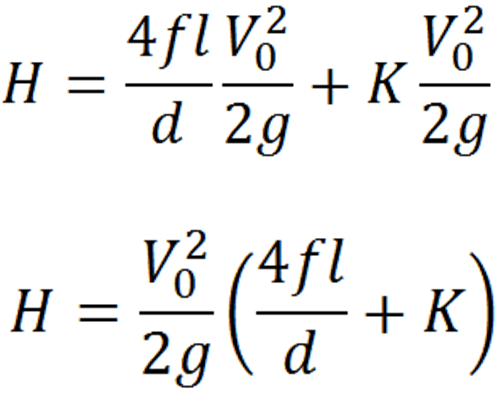Equation.5
• Comparing equations 4 and 5,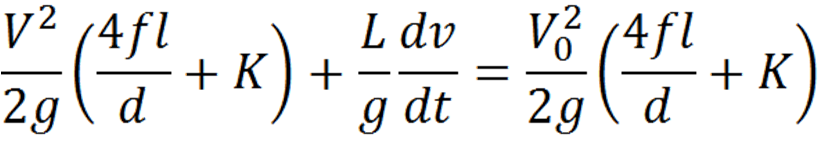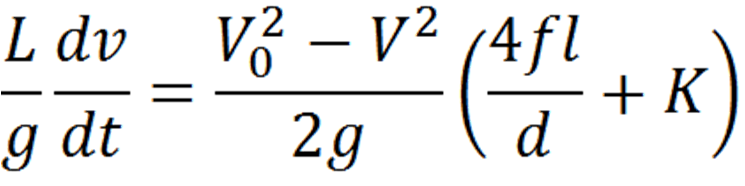Equation.6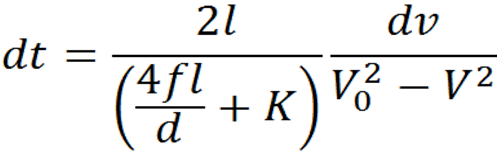Equation.7
• By integration,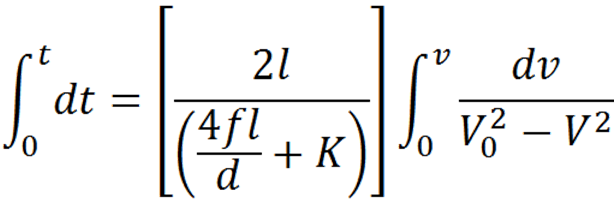Equation.8
• So the transient time is given by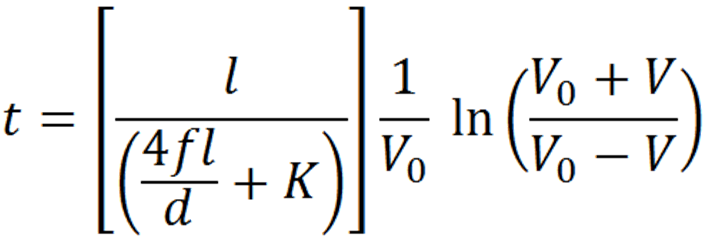Equation.9

[blogger]

Engineeersdaily

Name

Email *

Message *